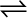7.19 A sample of pure PCl5 was introduced into an evacuated vessel at 473 K. After equilibrium was attained, concentration of PCl5 was found to be
0.5 × 10
–1 mol L–1. If value of Kc is 8.3 × 10–3, what are the concentrations of PCl3 and Cl2 at equilibrium?

PCl5 (g)PCl3 (g) + Cl2(g)

Let the concentrations of both  at equilibrium be x mol${\mathrm{L}}^{-1}$. The given reaction is:

It is given that of the value of the equilibrium constant, .

Now we can write the expression for equilibrium as:

Therefore, at equilibrium,